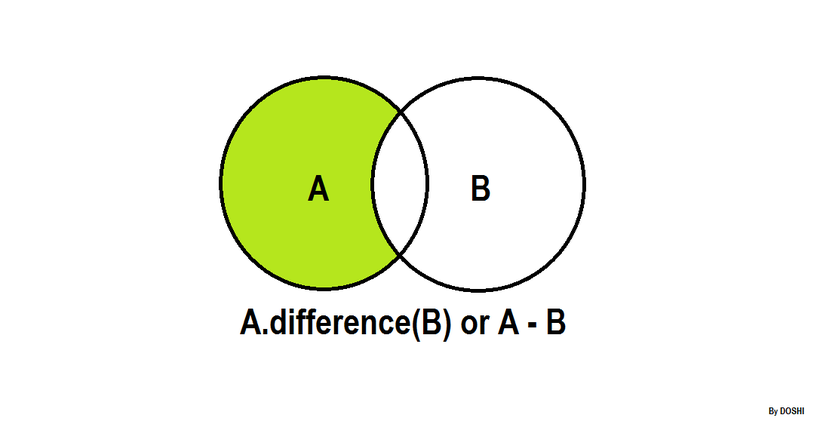## Algorithm

Problem Name: Python - Set .difference() Operation

In this HackerRank Functions in PYTHON problem solution,.difference()

The tool .difference() returns a set with all the elements from the set that are not in an iterable.
Sometimes the `-` operator is used in place of the .difference() tool, but it only operates on the set of elements in set.
Set is immutable to the .difference() operation (or the `-` operation).

``````>>> s = set("Hacker")
>>> print s.difference("Rank")
set(['c', 'r', 'e', 'H'])

>>> print s.difference(set(['R', 'a', 'n', 'k']))
set(['c', 'r', 'e', 'H'])

>>> print s.difference(['R', 'a', 'n', 'k'])
set(['c', 'r', 'e', 'H'])

>>> print s.difference(enumerate(['R', 'a', 'n', 'k']))
set(['a', 'c', 'r', 'e', 'H', 'k'])

>>> print s.difference({"Rank":1})
set(['a', 'c', 'e', 'H', 'k', 'r'])

>>> s - set("Rank")
set(['H', 'c', 'r', 'e'])
``````

Students of District College have a subscription to English and French newspapers. Some students have subscribed to only the English newspaper, some have subscribed to only the French newspaper, and some have subscribed to both newspapers.

You are given two sets of student roll numbers. One set has subscribed to the English newspaper, and one set has subscribed to the French newspaper. Your task is to find the total number of students who have subscribed to only English newspapers.

Input Format

The first line contains the number of students who have subscribed to the English newspaper.
The second line contains the space separated list of student roll numbers who have subscribed to the English newspaper.
The third line contains the number of students who have subscribed to the French newspaper.
The fourth line contains the space separated list of student roll numbers who have subscribed to the French newspaper.

Constraints

0 < Total number of students in college < 1000

Output Format

Output the total number of students who are subscribed to the English newspaper only.

Sample Input

``````9
1 2 3 4 5 6 7 8 9
9
10 1 2 3 11 21 55 6 8
``````

Sample Output

``````4
``````

Explanation

The roll numbers of students who only have English newspaper subscriptions are:

4, 5, 7, and 9.

Hence, the total is 4 students.

## Code Examples

### #1 Code Example with Python Programming

```Code - Python Programming```

``````
input()
s = set(input().split())
input()
print(len(s.difference(input().split())))
``````
Copy The Code &